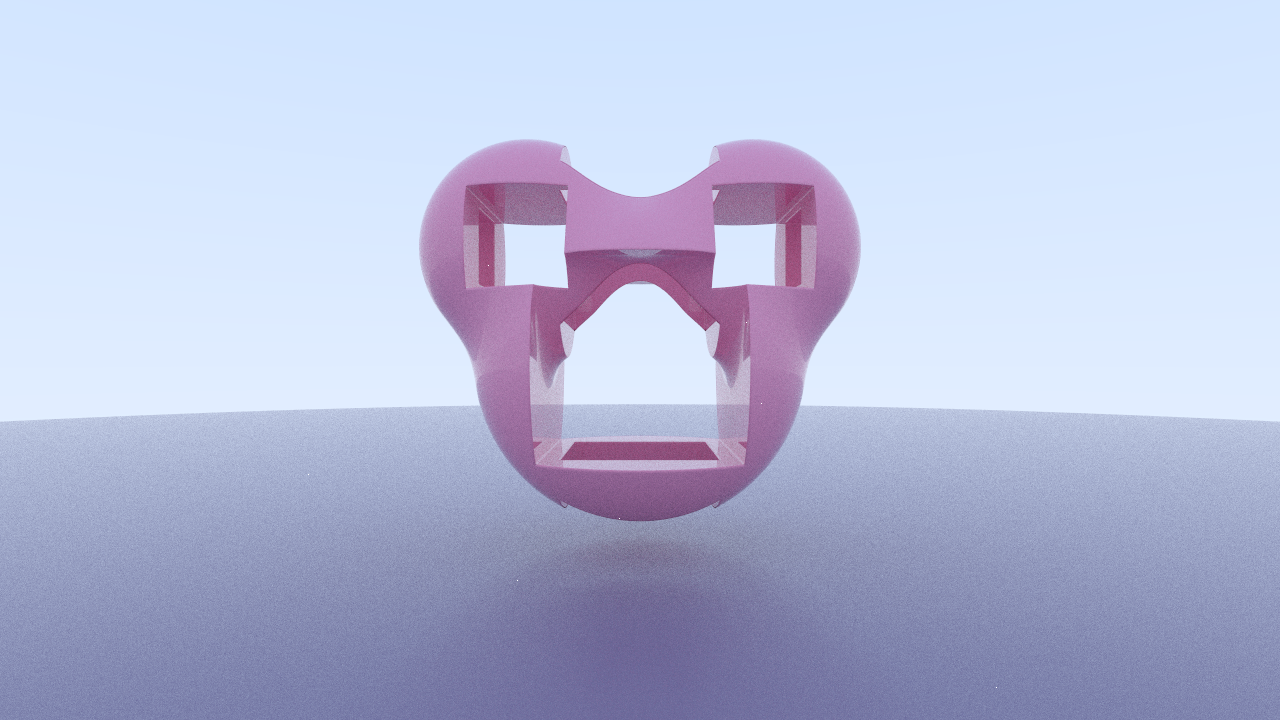# `sdfu` - Signed Distance Field Utilities

This is a small crate designed to help when working with signed distance fields in the context of computer graphics, especially ray-marching based renderers. Most of what is here is based on Inigo Quilez' excellent articles.

If you're using one of the more popular math libraries in Rust, then just enable the corresponding feature and hopefully all the necessary traits are already implemented for you so that you can just start passing in your `Vec3`s or whatever your lib calls them and you're off to the races! If not, then you can implement the necessary traits in the `mathtypes` module and still use this library with your own math lib.

This crate is built around the central trait `SDF`. This trait is structured in a similar way to how `std::iter::Iterator` works. Anything that implements `SDF` is able to return a distance from a point to its distance field. SDFs can be combined, modified, and otherwise used for various tasks by using the combinator methods on the `SDF` trait, or by directly using the structs that actually implement those combinators.

Most `SDF`s will be build up from one or more primitives being modified and combined together--the distance fields in the `primitive` module provide good starting points for this.

# DemoThe image above was rendered with my own path tracing renderer, `rayn`, by leveraging `sdfu`. The SDF that is rendered above was created with the following code:

```use sdfu::SDF;

let sdf = sdfu::Sphere::new(0.45)
.subtract(
sdfu::Box::new(Vec3::new(0.25, 0.25, 1.5)))
.union_smooth(
sdfu::Sphere::new(0.3).translate(Vec3::new(0.3, 0.3, 0.0)),
0.1)
.union_smooth(
sdfu::Sphere::new(0.3).translate(Vec3::new(-0.3, 0.3, 0.0)),
0.1)
.subtract(
sdfu::Box::new(Vec3::new(0.125, 0.125, 1.5)).translate(Vec3::new(-0.3, 0.3, 0.0)))
.subtract(
sdfu::Box::new(Vec3::new(0.125, 0.125, 1.5)).translate(Vec3::new(0.3, 0.3, 0.0)))
.subtract(
sdfu::Box::new(Vec3::new(1.5, 0.1, 0.1)).translate(Vec3::new(0.0, 0.3, 0.0)))
.subtract(
sdfu::Box::new(Vec3::new(0.2, 2.0, 0.2)))
.translate(Vec3::new(0.0, 0.0, -1.0));```

## Re-exports

 `pub use mathtypes::Dim2D;` `pub use mathtypes::Dim3D;` `pub use mathtypes::Dimension;` `pub use primitives::*;`

## Modules

 mathtypes mods Modifiers for SDFs. ops Operations you can perform to combine two SDFs. primitives A collection of primitive SDFs that may the modified using functions in `mods` or combined using functions in `ops`. Note that these primitives are always centered around the origin and that you must transform the point you are sampling into 'primitive-local' space. Functions are provided in `mods` to do this easier. util Other random utilities that are helpful when using SDFs in computer graphics applications, such as estimating normals.

## Traits

 SDF The core trait of this crate; an implementor of this trait is able to take in a vector and return the min distance from that vector to a distance field.